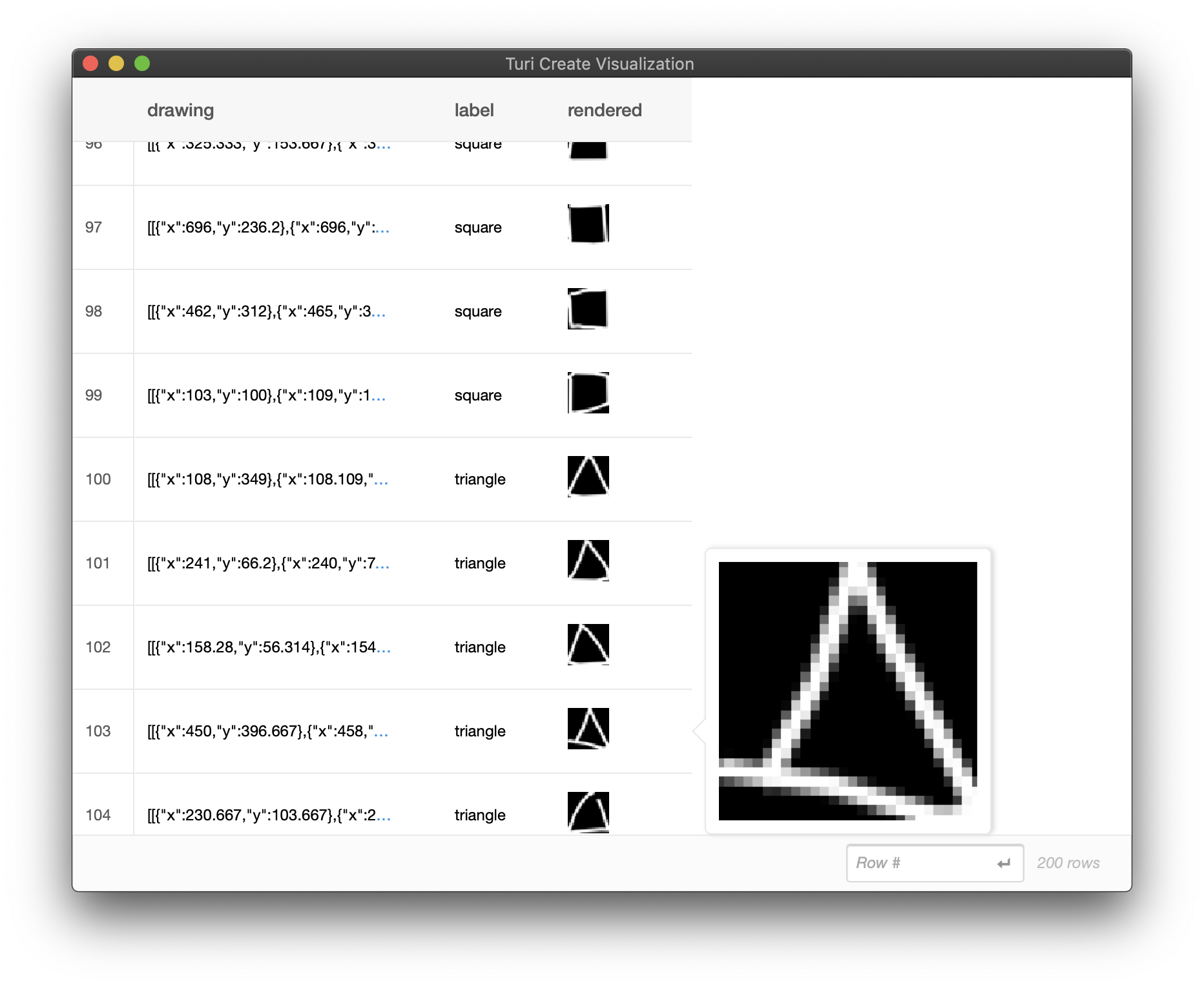# Quick, Draw! Data Preparation

In this section, we will setup a subset of Quick, Draw!1. Our goal is to make a drawing classifier for squares and triangles.

Note: Requires Turi Create 5.4 or above

We start by dowloading a few examples of "square" and "triangle" shapes -- around 120,000 examples each, both as bitmaps and as stroke-based drawings. The following script (run from your terminal) should get you started.

# Make directories with the data
mkdir quickdraw
cd quickdraw
mkdir bitmaps strokes sframes

cd bitmaps

# Bitmap data (around 90 MB each)

cd ../strokes

# Stroke data (around 200 MB each)

cd ../..

After running the above script, you should have a directory structure like this:

quickdraw/
bitmaps/
square.npy
triangle.npy
strokes/
square.ndjson
triangle.ndjson
sframes/


For both bitmap and stroke-based drawing input formats, we will sample 100 examples from each of the classes and turn it into an SFrame.

#### Using Bitmap Data

Here is a snippet to sample 100 examples per class into an SFrame for bitmap data as the input format:

import turicreate as tc
import numpy as np
import os

random_state = np.random.RandomState(100)

# Change if applicable
quickdraw_dir = 'quickdraw'
bitmaps_dir = os.path.join(quickdraw_dir, 'bitmaps')
sframes_dir = os.path.join(quickdraw_dir, 'sframes')
npy_ext = '.npy'
num_examples_per_class = 100
classes = ["square", "triangle"]
num_classes = len(classes)

def build_bitmap_sframe():
bitmaps_list, labels_list = [], []
for class_name in classes:
class_data = np.load(os.path.join(bitmaps_dir, class_name + npy_ext))
random_state.shuffle(class_data)
class_data_selected = class_data[:num_examples_per_class]
class_data_selected = class_data_selected.reshape(
class_data_selected.shape, 28, 28, 1)
for np_pixel_data in class_data_selected:
FORMAT_RAW = 2
bitmap = tc.Image(_image_data = np_pixel_data.tobytes(),
_width = np_pixel_data.shape,
_height = np_pixel_data.shape,
_channels = np_pixel_data.shape,
_format_enum = FORMAT_RAW,
_image_data_size = np_pixel_data.size)
bitmaps_list.append(bitmap)
labels_list.append(class_name)

sf = tc.SFrame({"drawing": bitmaps_list, "label": labels_list})
sf.save(os.path.join(sframes_dir, "bitmap_square_triangle.sframe"))
return sf

sf = build_bitmap_sframe()

After building the two SFrames, your directory structure should look like the following:

quickdraw/
bitmaps/
square.npy
triangle.npy
strokes/
square.ndjson
triangle.ndjson
sframes/
bitmap_square_triangle.sframe
stroke_square_triangle.sframe

#### Using Stroke-Based Drawing Data

For stroke-based drawing data to be given as the feature in the input SFrame to turicreate.drawing_classifier.create, the stroke-based drawing data must adhere to the following format:

Each drawing must be represented as a list of strokes, where each stroke must be represented as a list of points in the order that they were drawn. Each point must be represented as a dictionary with exactly two keys, "x" and "y", the values of which must be numerical, i.e. integer or float. Here is an example of a drawing with two strokes that have five points each:

example_drawing = [
[
{"x": 1.0, "y": 2.0},
{"x": 2.0, "y": 2.0},
{"x": 3.0, "y": 2.0},
{"x": 4.0, "y": 2.0},
{"x": 5.0, "y": 2.0}
], # end of first stroke
[
{"x": 10.0, "y": 10.0},
{"x": 10.5, "y": 10.5},
{"x": 11.0, "y": 11.0},
{"x": 12.5, "y": 12.5},
{"x": 15.0, "y": 15.0}
]
]

Our first task at hand is to build an SFrame that adheres to this format from the "Quick, Draw!" data that we have downloaded. Here is a snippet to accomplish that:

import turicreate as tc
import numpy as np
import os
import json

random_state = np.random.RandomState(100)

# Change if applicable
quickdraw_dir = 'quickdraw'
strokes_dir = os.path.join(quickdraw_dir, 'strokes')
sframes_dir = os.path.join(quickdraw_dir, 'sframes')
ndjson_ext = '.ndjson'
num_examples_per_class = 100
classes = ["square", "triangle"]
num_classes = len(classes)

def build_strokes_sframe():
drawings_list, labels_list = [], []
for class_name in classes:
with open(os.path.join(strokes_dir, class_name+ndjson_ext)) as fin:
ndjson_data = list(map(lambda x: x.strip(), fin.readlines()))
random_state.shuffle(ndjson_data)
raw_drawing_list = [ndjson["drawing"] for ndjson in ndjson_data_selected]
def raw_to_final(raw_drawing):
return [
[
{
"x": raw_drawing[stroke_id][i],
"y": raw_drawing[stroke_id][i]
} for i in range(len(raw_drawing[stroke_id]))
]
for stroke_id in range(len(raw_drawing))
]

final_drawing_list = list(map(raw_to_final, raw_drawing_list))
drawings_list.extend(final_drawing_list)
labels_list.extend([class_name] * num_examples_per_class)
sf = tc.SFrame({"drawing": drawings_list, "label": labels_list})
sf.save(os.path.join(sframes_dir, "stroke_square_triangle.sframe"))
return sf

sf = build_strokes_sframe()

When stroke-based drawing data is given as input to the Drawing Classifier Toolkit either at train or inference time, the toolkit converts the stroke-based drawings into bitmaps as part of preprocessing so the Neural Network can consume them. See How it works! for more information about the preprocessing done under the hood.

To visualize what your stroke-based drawings look like when rendered as a bitmap, you can run the following utility function:

sf = build_strokes_sframe()
sf["rendered"] = tc.drawing_classifier.util.draw_strokes(sf["drawing"])
sf.explore()